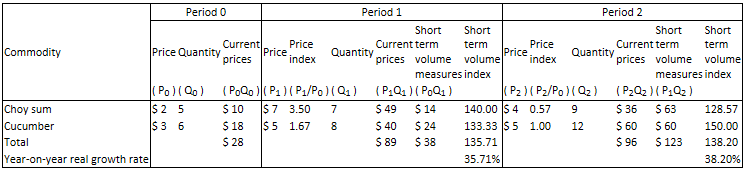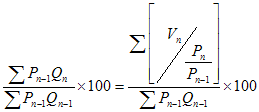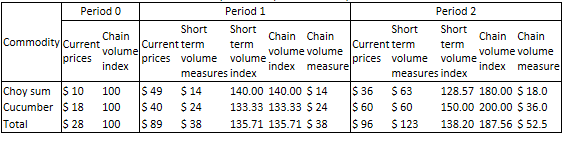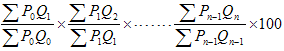Continuing with our example of choy sum and cucumber, calculation of chain volume measures are illustrated: Table 2. Short term volume measures and short term volume indicesValue of production at current prices is the same as in Section II. There are 2 steps for calculating chain volume measures. First, calculate the short term volume measures and short term volume indices, which are volume measures and volume indices in comparison with the preceding year. Second, compound the short term volume indices to produce a time series of chain volume index and chain volume measures in terms of the price of the reference year. Detailed mechanism and steps are illustrated by the abovementioned example as follows:

Volume indices in Step 1 are derived with similar calculation as volume index of constant price estimates; the only difference is volume measures are valued at the prices of choy sum and cucumber in the preceding year. Divide short term volume measures by the current prices in the preceding year and multiply by 100 to derive the short term volume indices. The formula of short term volume index is:Table 2 shows that the short term volume measures of choy sum and cucumber in Period 1 are \$14 (multiply the quantity of choy sum in Period 1 (7) by the price of choy sum in Period 0 (\$2)) and \$24 respectively, and the total short term volume measures is \$38. The short term volume measures of choy sum and cucumber in Period 2 are \$63 and \$60 respectively, and the total short term volume measure is \$123. Divide the short term volume measures of the total and each component (choy sum and cucumber) by the respective value of total and each component at current prices in the preceding year and multiply by 100 to derive the short term volume indices of the total and each component in the current year. Deduct 100 from the short term volume indices and divide by 100 to derive the real growth between 2 consecutive years. Short term volume indices of choy sum and cucumber in Period 1 are 140.00 and 133.33 respectively; the short term volume index of the total is 135.71; the real growth of choy sum, cucumber and the total is 40.00%, 33.33% and 35.71% respectively. Short term volume indices of choy sum and cucumber in Period 2 are 128.57 and 150.00 respectively; the short term volume index of the total is 138.20; the real growth of choy sum, cucumber and the total is 28.57%, 50.00% and 38.20% respectively. Annual short term volume measures and indices must be calculated individually.

Table 3. Chain volume measures and indices (reference year = Period 0)Individual short term volume indices and real growth between 2 years are calculated in Step 1. In order to derive the chain volume indices for 3 years or above, it is necessary to multiply the individual short term volume indices and this process is called chain-linking. The mechanism is to select a reference year, where the chain volume indices equal 100; the chain volume indices of other years are derived by dividing the short term volume indices by 100, then multiply the index of each year to the reference year. Chain-linking for choy sum, cucumber and the total are done individually, as follows:Table 3 shows that as Period 1 is the year following the reference year, the chain volume index of choy sum equals its short term volume index (140.00), whereas the chain volume index in Period 2 is 180, i.e. multiply the short term volume index (128.57) by the short term volume index in Period 1 (140.00) and divide by 100.

Chain volume measure is derived by dividing the chain volume index by 100 and multiply by the current price in the reference year. Table 3 shows that chain volume measure (reference year = Period 0) of choy sum in Period 1 is \$14, i.e. divide chain volume index of choy sum in Period 1 (140.00) by 100, then multiply by the current price of choy sum in Period 0 (\$10). Similarly, chain volume index and chain volume measure of cucumber in Period 1 are 133.33 and \$24 respectively. Chain volume index and chain volume measure of the total in Period 1 are 135.71 and \$38 respectively. Chain volume measure of choy sum in Period 2 is \$18, i.e. divide chain volume index of choy sum in Period 1 (180.00) by 100, then multiply by the current price of choy sum in Period 0 (\$10). Chain volume index and chain volume measure of cucumber in Period 1 are 200.00 and \$36 respectively. Chain volume index and chain volume measure of the total in Period 1 are 187.56 and \$52.5 respectively.